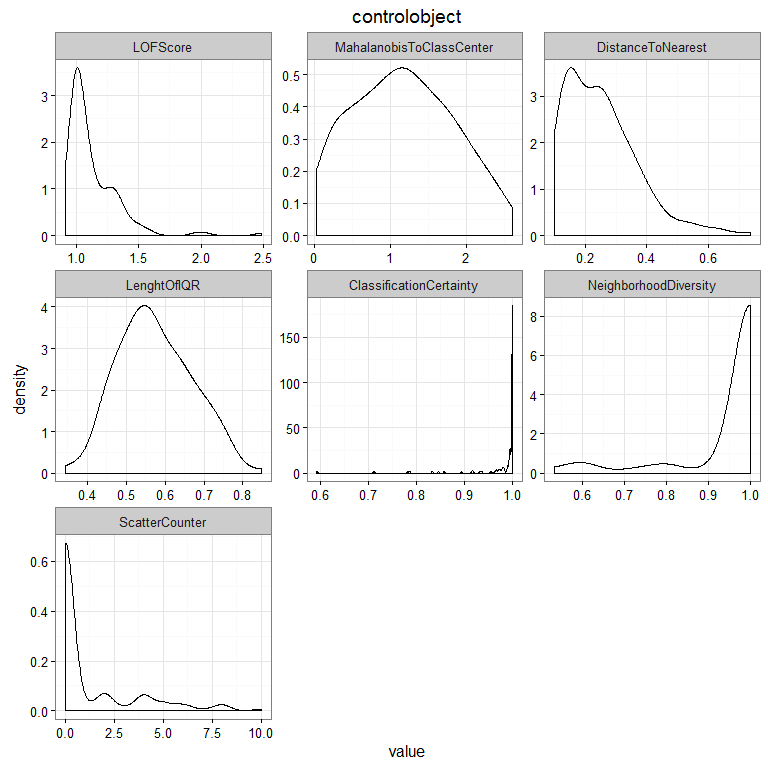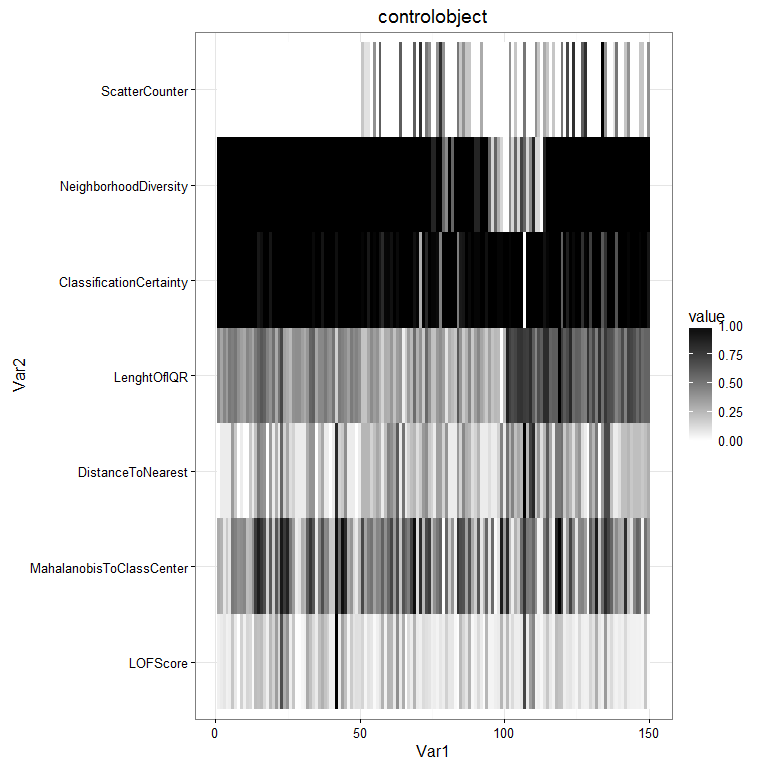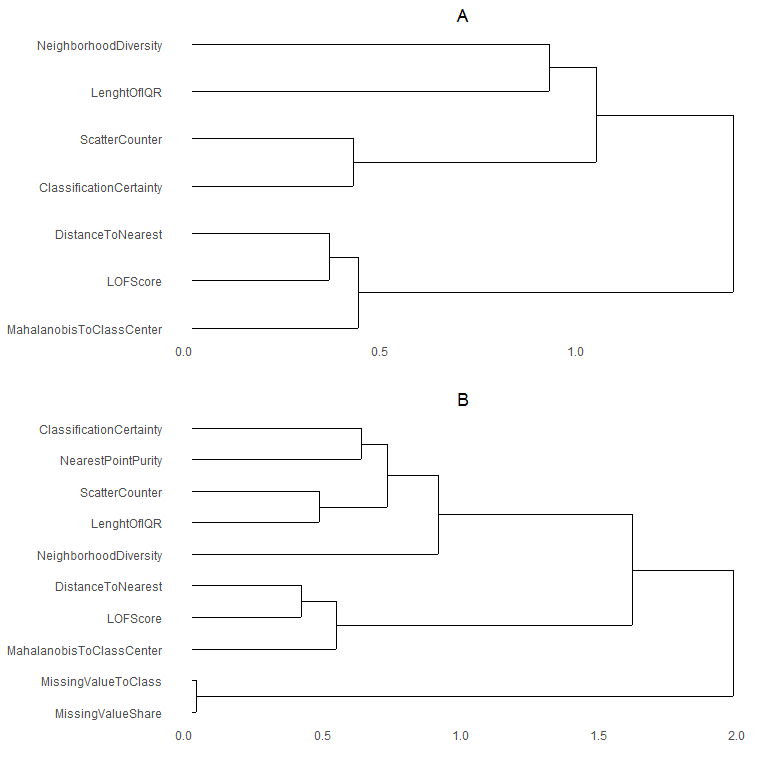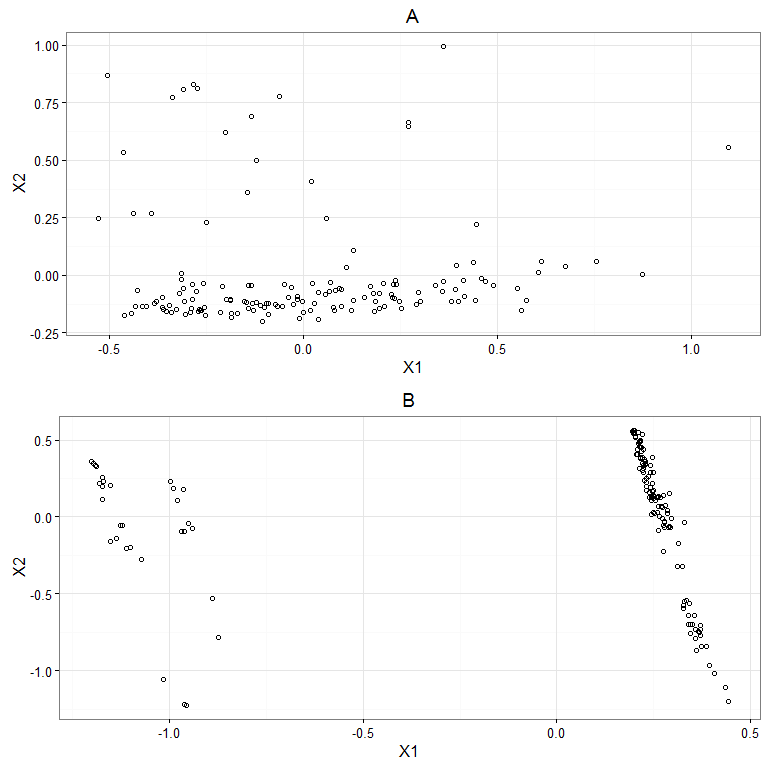# Preproviz

#### 2016-07-09

Data quality issues such as missing values and outliers are often interdependent, which makes preprocessing both time-consuming and leads to suboptimal performance in knowledge discovery tasks. This package supports preprocessing decision making by visualizing interdependent data quality issues through means of feature construction. The user can define his own application domain specific constructed features that express the quality of a data point such as number of missing values in the point or use nine default features. The outcome can be explored with plot methods and the feature constructed data acquired with get methods.

## Quick start

Simple exploration can be done by passing a data frame as an argument. The data frame must have one factor variable and other variables numeric.

library(preproviz)
result <- preproviz(iris)
##  "Data in process: controlobject"

The resulting object can be plotted with various plot functions.

plotDENSITY(result)plotHEATMAP(result)## Comparisons

The package supports comparison of multiple data sets or different versions of a same data set.

Let’s make some test data.

iris2 <- iris
iris2[sample(1:150,30), 1] <- NA # adding missing values
iris2[sample(1:150,30), 5] <- levels(iris2\$Species) # adding inconsistency 

and then setup comparison between iris and iris2.

result <- preproviz(list(iris, iris2))
##  "Data in process: A"
##  "Data in process: B"

Plotting how the constructed features cluster (that is, which features are linearly dependent on each other).

plotVARCLUST(result)and then how the constructed feature data points cluster when reduced to two dimensions.

plotCMDS(result)## Customization

Finally, the setups can be customized in detail:

customparameters <- initializeparameterclassobject(list("LOFScore", "ScatterCounter"))
setup1 <- initializesetupclassobject("setup1", customparameters, initializedataobject(iris))
setup2 <- initializesetupclassobject("setup2", customparameters, initializedataobject(otherdataframehere))
control <- initializecontrolclassobject(list("setup1", "setup2"))
result <- preproviz(control)

and new constructe features added to the system:

constructfeature("MissingValueShare", "apply(data, 1, function(x) sum(is.na(x))/ncol(data))", impute=TRUE)

## Default contructed features

There are nine default constructed features:

• MissingValueShare, count the number of missing values on a row and divide it by the total number of features

• LenghtOfIQR, min-max normalize the data and compute the length of IQR for each row (i.e. flatness of reply)

• DistanceToNearest, min-max normalize the data and compute the Euclidean distance to the nearest data point

• ClassificationCentainty, compute the random forest class propability that has the highest value

• ClassificationScatter. compute the scatter counter (number of changes in class labels) in 1/10 point neighbourhood

• NearestPointPurity,Neareast point is of same class or not

• NeighborhoodDiversity, count the number of dominant class points in 1/10 data set size neighborhood and divide it with the total number of classes in the data set.

• LOFScore, compute LOF scores

• MahalanobisDistance, compute Mahalanobis distance to class center

• ClusteringTendency, Count the Hopkins statistic without a row and divide it with Hopkings statistic for all rows